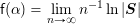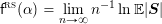# Research Vignette: Phase Transitions in Random Constraint Satisfaction Problems

by Allan Sly and Nike Sun

## Random constraint satisfaction problems

Suppose we are given a finite graph. Does it admit a proper three-coloring? This is one example of a constraint satisfaction problem (CSP). Generally speaking, a CSP is specified by a list of variables, subject to a set of constraints. A prototypical computational question is to decide, in a given CSP instance, whether there exists any variable assignment satisfying all the constraints. If yes, then one may further ask how many solutions are there, or what is the optimal value of some objective function over the set of solutions.

On general inputs, these questions are not believed to be computationally tractable — many CSPs are NP-complete. For the problem of deciding whether a given graph is three-colorable, the best known algorithms require more than 2 time on the “worst” graphs of size n. In view of this, it became natural to develop notions of tractability in “average” or “typical” cases (Levin 1986). One of the standard ways to approach this has been via ensembles of random CSPs. For example, say that we sample a large random graph from some natural distribution — might it be the case that “in most cases” it is easy to decide whether the graph is three-colorable?

## Satisfiability transitions in random CSPs

For all the commonly studied random CSP ensembles, the (average-case) complexity remains an open question. A possible reason for this is that even before going into computational considerations, there are far more basic properties of random CSPs that have not been resolved. For example, it seems very difficult to estimate the probability that a random CSP is satisfiable. In many examples, the random CSP can be naturally parametrized by a “constraint level” α — commonly, α is the ratio of the number of constraints to the number of variables. Numerical experiments (early 1990s) indicated that some of the most canonical random CSPs have a sharp satisfiability transition — a critical threshold 0 < αsat < ∞ such that the random CSP is satisfiable with high probability for α < αsat, and unsatisfiable with high probability for α > αsat.

This transition was most studied for the random k-SAT problem, defined as follows. Start with a set of boolean variables x1,…,xn. A literal is a variable xi, or its negation ¬xi. For i ≥ 1, the random clause ai is the disjunction of k literals chosen independently and uniformly from the set of literals {x1x1,…,xnxn}; the clauses are chosen mutually independently. A random k-SAT formula at density α is defined by the conjunction of the first M clauses where M is a Poisson() random variable: Φ = a1 ∧ … ∧ aM. Its solution space is the set S = S(Φ) of variable assignments x ∈ {T,F}n which make the formula Φ satisfied, meaning Φ(x) = T. Let p = pk(α,n) be the probability that the random formula Φ is satisfiable — equivalently, p is the probability for S to be nonempty. The satisfiability threshold conjecture is that for each k ≥ 2, there is a critical αsat (depending on k but not on n) such that

This was proved for k = 2 (with αsat = 1) in a few independent works around 1992 (Chvátal–Reed, de la Vega, Goerdt), but remained open for k ≥ 3. It is known (Friedgut 1999) that for each k ≥ 3 there exists a sharp threshold sequence αsat(n) — the conjecture holds if the sequence converges.

## Statistical physics of random CSPs

Since the 1980s, these questions have also attracted the attention of statistical physicists, who observed that random CSPs can be viewed in the framework of spin glasses (modeling the behavior of certain magnetic alloys). The most classical model of this type is the SK spin glass (Sherrington–Kirkpatrick 1975), which features spins x1,…,xn ∈ {−,+} with random (Gaussian) interactions gij. An asymptotic characterization of the SK model was proposed by Parisi in a series of seminal papers (around 1980). Key aspects of this conjecture were rigorously proved in the past decade (Guerra 2003, Talagrand 2006, Panchenko 2012); although important open questions remain. The constraints (clauses) of the random k-SAT model are analogous to the interactions gij of the SK model.

A major difference between these models is that the SK spin glass is defined on a complete graph — there is an interaction gij for every pair (i,j) — whereas many random CSPs, k-SAT included, are more interesting in the sparse regime, where every variable participates in a bounded number of interactions. In spite of this, physicists conjectured an asymptotic characterization of random k-SAT which bears vague resemblances to the Parisi solution for SK. The full conjecture is remarkably detailed and appears in Krzakala et al. 2007; some aspects of it are hinted at in various earlier papers (Mézard, Mertens, Parisi, Zecchina). Among other implications, the conjecture yields explicit formulas for two thresholds: one is the satisfiability threshold αsat, and the other is the condensation threshold αcond ∈ (0,αsat). For α < αcond, the measure ν has correlation decay — if i and j are far apart in the k-SAT interaction graph, then xi and xj are roughly independent under ν. For α > αcond this no longer holds, meaning that ν has non-negligible long-range correlations.

## Exact thresholds and partition function asymptotics

A key insight emerging from the statistical physics literature is that the long-range correlations above αcond are precisely what make it difficult to pin down αsat. Standard probabilistic techniques (in particular, the moment method) implicitly take advantage of some kind of correlation decay in the measure. In fact, the physics predictions for αcond and αsat are based on assuming correlation decay, not for ν itself, but for a family of measures obtained by tilting ν in a certain way. The assumption is supported by numerical evidence and heuristic arguments, but has been lacking a mathematical theory.

In recent years there has been some progress in rigorously defining and analyzing some versions of these tilted measures. This led to the rigorous determination of αsat in some models, including a proof the k-SAT threshold conjecture for large k (Ding–Sly–Sun 2014). The exact value of αcond has been rigorously established for random (Erdős–Rényi) graph coloring with a large number of colors (Bapst et al. 2014). During the recent Simons program, we studied the asymptotics of the partition function (number of solutions) in the random regular k-NAE-SAT model. This model simplifies random k-SAT in two ways: (i) every variable participates in the same number of clauses, and (ii) it requires both the assignment and its negation to satisfy the formula. For this model we determined the free energyas a function of α (Sly–Sun–Zhang 2016). For comparison, the “annealed” free energyis easy to compute, and is simply linearly decreasing in α. The free energy f agrees with fRS up to αcond, and diverges thereafter. The main difficulty is to compute f above αcond, which is done via a certain tilt of the original measure.

We point to some references below for further details, and many open problems.

On the Sherrington–Kirkpatrick model:

• D. Sherrington and S. Kirkpatrick. “Solvable model of a spin-glass.” Phys. Rev. Lett. 35:26 (1975), 1792-1795.
• G. Parisi. “Order parameter for spin-glasses.” Phys. Rev. Lett. 50:24 (1983), 1946-1948.
• F. Guerra. “Broken replica symmetry bounds in the mean field spin glass model.” Comm. Math. Phys. 233:1 (2003), 1–12.
• M. Talagrand. “The Parisi formula.” Ann. Math. 163:1 (2006), 221-263.
• D. Panchenko. “The Parisi ultrametricity conjecture.” Ann. Math. 177:1 (2013), 383-393.

Physics predictions for sparse random CSPs:

• M. Mézard, G. Parisi, and R. Zecchina. “Analytic and algorithmic solution of random satisfiability problems.” Science 297:5582 (2002), 812-815.
• S. Mertens, M. Mézard, and R. Zecchina. “Threshold values of random k-SAT from the cavity method.” Random Struct. Algor. 28:3 (2006), 340-373.
• F. Krzakala, A. Montanari, F. Ricci-Tersenghi, G. Semerjian, and L. Zdeborová. “Gibbs states and the set of solutions of random constraint satisfaction problems.” Proc. Natl. Acad. Sci. 104:25 (2007), 10318-10323.
• M. Mézard and A. Montanari. Information, physics, and computation. Oxford University Press (2009).

Recent rigorous results for sparse random CSPs:

• V. Bapst, A. Coja-Oghlan, S. Hetterich, F. Raßmann, and D. Vilenchik. “The condensation phase transition in random graph coloring.” Proc. 18th RANDOM (2014)Comm. Math. Phys. 341:2 (2016), 543-606.
• J. Ding, A. Sly, and N. Sun. “Proof of the satisfiability conjecture for large k.” Proc. 47th STOC (2015).
• A. Sly, N. Sun, and Y. Zhang. “The number of solutions for random regular NAE-SAT.” Proc. 57th FOCS (2016).

Other works cited:

• L. Levin. “Average case complete problems.” SIAM J. Comput. 15:1 (1986), 285-286.
• V. Chvátal and B. Reed. “Mick gets some (the odds are on his side) [satisfiability].” Proc. 33rd FOCS (1992).
• A. Goerdt. “A threshold for unsatisfiability.” Proc. 17th MFCS (1992).
• F. de la Vega. “On random 2-SAT.” Unpublished manuscript (1992).
• F. de la Vega. “Random 2-SAT: results and problems.” Theor. Comput. Sci., 265:1 (2001), 131-146.
• E. Friedgut. “Sharp thresholds of graph properties, and the k-SAT problem.” With an appendix by J. Bourgain. J. Amer. Math. Soc. 12:4 (1999), 1017-1054.

This site uses Akismet to reduce spam. Learn how your comment data is processed.